## 【LeetCode热题100】打卡第44天：倒数第30~25题

article2023/10/3 19:41:58/

### 文章目录

• 【LeetCode热题100】打卡第44天：倒数第30~25题
• ⛅前言
• 移动零
• 🔒题目
• 🔑题解
• 寻找重复数
• 🔒题目
• 🔑题解
• 二叉树的序列化与反序列化
• 🔒题目
• 🔑题解
• 最长递增子序列
• 🔒题目
• 🔑题解
• 删除无效括号
• 🔒题目
• 🔑题解

# 【LeetCode热题100】打卡第44天：倒数第30~25题

## ⛅前言

LeetCode热题100专栏🚀：LeetCode热题100

Gitee地址📁：知识汲取者 (aghp) - Gitee.com

PS：作者水平有限，如有错误或描述不当的地方，恳请及时告诉作者，作者将不胜感激

# 移动零

## 🔒题目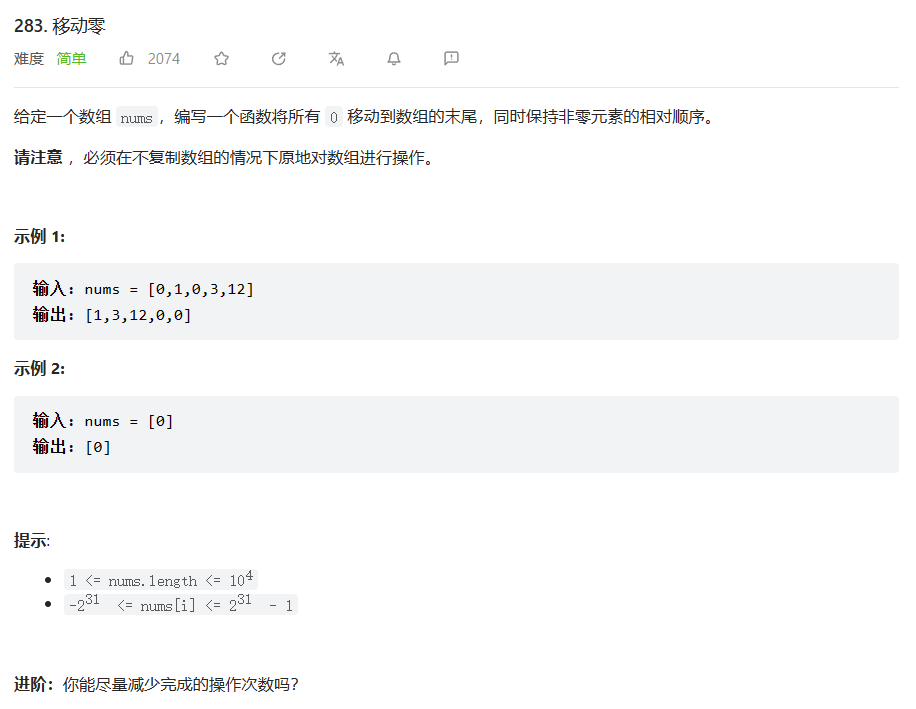## 🔑题解

• 解法一：暴力枚举即可

但是我们使用copyOfRange方法存在一个弊端，它会重现创建一个数组，然后将值赋值给新的数组引用，给不是在原有的数组引用上进行赋值，所以这里就导致最终无法修改到我们要实现效果的数组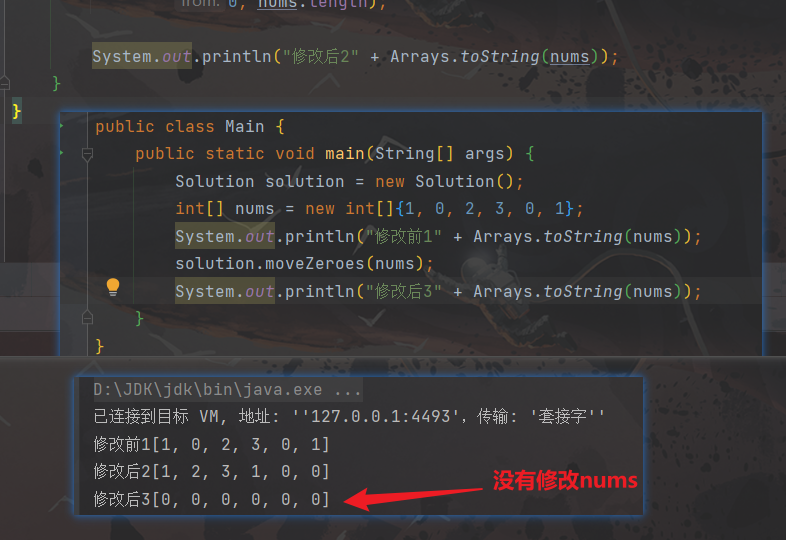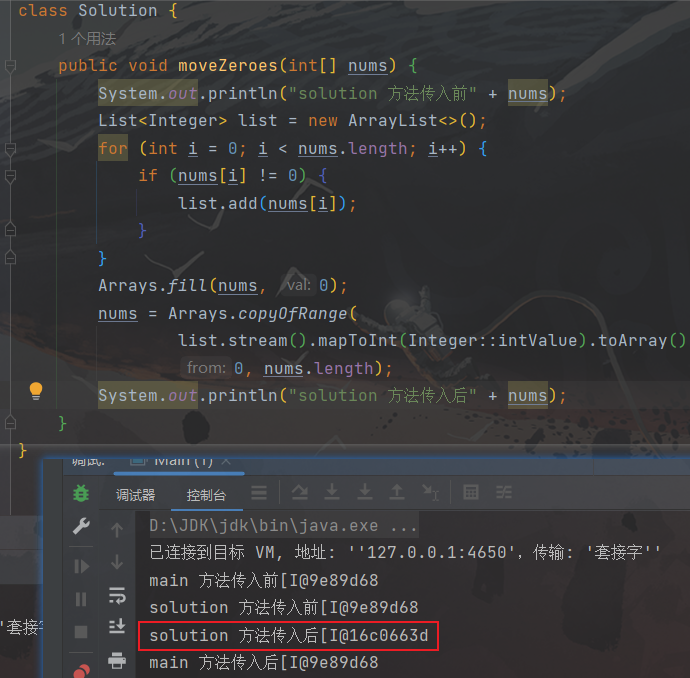下方代码，最终输出的nums全部是 0

/**
* @author ghp
* @title
* @description
*/
class Solution {
public void moveZeroes(int[] nums) {
List<Integer> list = new ArrayList<>();
for (int i = 0; i < nums.length; i++) {
if (nums[i] != 0){
}
}
Arrays.fill(nums, 0);
nums =  Arrays.copyOfRange(
list.stream().mapToInt(Integer::intValue).toArray(),
0, nums.length);
}
}


复杂度分析：

• 时间复杂度： O ( n ) O(n)
• 空间复杂度： O ( n ) O(n)

其中 n n 为数组中元素的个数

解决方法：使用for循环，逐个赋值（这里我就是使用lambda表达式实现，效果都是一样的，但是这种更加优雅）

/**
* @author ghp
* @title
* @description
*/
class Solution {
public void moveZeroes(int[] nums) {
List<Integer> list = new ArrayList<>();
for (int i = 0; i < nums.length; i++) {
if (nums[i] != 0) {
}
}
Arrays.fill(nums, 0);
IntStream.range(0, list.size())
.forEach(i -> nums[i] = list.get(i));
}
}

• 解法二：双指针

这个思路是非类似于快排的那个划分左右区间，设置两个指针，使得左区间都比主元小，右区间都比主元大或等。

这里我们相当于是把0当作主元，左区间都是不等于0的，右区间都是等于0的

class Solution {
public void moveZeroes(int[] nums) {
int i = 0;
// 遍历数组，将非0元素放到i的左侧
for (int j = 0; j < nums.length; j++) {
if (nums[j] != 0){
// 当前元素不等于0，将非0元素放到i的左侧
int t = nums[j];
nums[j] = nums[i];
nums[i] = t;
i++;
}
}
}
}


复杂度分析：

• 时间复杂度： O ( n ) O(n)
• 空间复杂度： O ( 1 ) O(1)

其中 n n 为数组中元素的个数

# 寻找重复数

## 🔒题目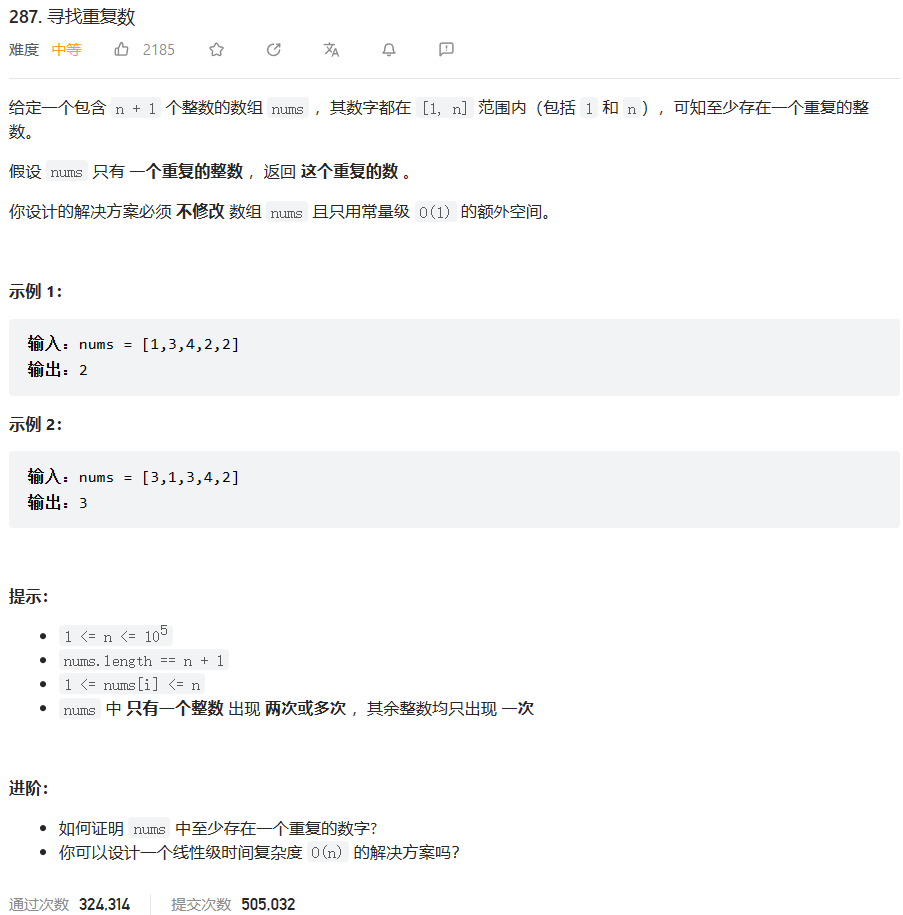## 🔑题解

1. 需要额外空间，需要修改原始数组：排序

2. 需要额外空间，不需要修改原始数组：计数法、哈希表

3. 不需要额外空间，需要修改原始数组：标记法、索引排序

4. 不需要额外空间，不需要修改原始数组：暴力枚举、二分查找、位运算、快慢指针

PS：本文只讲解了二分查找、快慢指针、位运算三种能过且比较牛的方法，关于其它方法感兴趣都可以参考这篇文章：9种方法（可能是目前最全的），拓展大家思路 - 寻找重复数 - 力扣（LeetCode）

• 解法一：快慢指针（Floyd 判圈算法）

这个算法在前面已经多次遇到了，比如：第33天的环形链表、第34天的排序链表、第35天的相交链表、第40天的回文链表等都能看到快慢指针算法的身影。可能我们一下子无法直接联想到环形链表，这里我们画一个草图，将数组转换成一个环形链表（这是一种数学抽象，类似于七桥问题，把一个问题抽象成另一个与之等价的问题）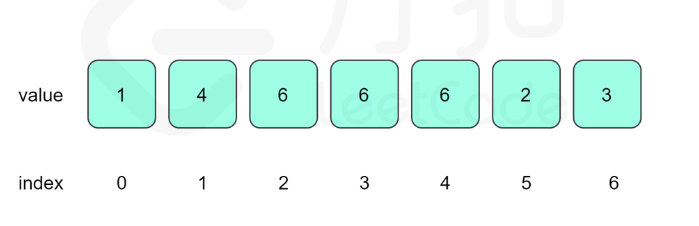我们把数值的值当成链表的下一个节点，这个值与索引进行一个映射，从而可以通过上面的链表得到下面这个链表，此时我们把”要数组中的找重复元素“这个问题转换成"要找链表中环的入口节点"，说到这里，如果你对环形链表这一题有经验的话，很快就能够解决了。如果你对环形链表不是很懂的话，可以参考这篇文章【LeetCode热题100】打卡第33天：环形链表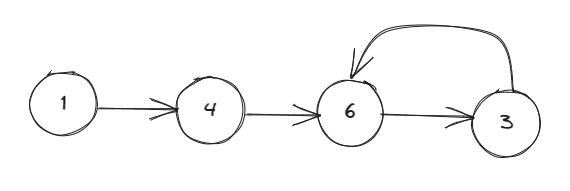注意：本题能够使用快慢指针的前提是 1 < = n u m s [ i ] < = n 1<=nums[i]<=n ，这样能够保障指针无论如何移动都不会出现索引越界

这里初略讲解以下如何定位环形链表的入环节点：

1. 第一次遍历，fast比slow多走一步，寻找到fast和slow相等的节点，然后将fast重置到起始节点
2. 第二次遍历，fast和slow走相同的步数，寻找到fast和slow相等的节点，此时fast和slow相遇的节点就是入环节点

至于详细证明思路，可以参考我上面给出的那个链接，链接的那篇文章中已给出比较详细的解答了

/**
* @author ghp
* @title
* @description
*/
class Solution {
public int findDuplicate(int[] nums) {
int fast = 0, slow = 0;
do {
fast = nums[nums[fast]];
slow = nums[slow];
} while (fast != slow);
fast = 0;
while (fast != slow) {
fast = nums[fast];
slow = nums[slow];
}
return fast;
}
}


复杂度分析：

• 时间复杂度： O ( n ) O(n)
• 空间复杂度： O ( 1 ) O(1)

其中n为数组中元素的个数

• 解法二：二分查找

本题主要用到了抽屉原理，简单来说就是把 10 个苹果放进 9 个抽屉，至少有一个抽屉里至少放 2 个苹果。

其此我们还需要寻找出有序的地方，本题有序的地方是隐式的，即比当前元素小的元素是有序的，只要发现这一点，其实就会变得很简单，但往往这一点一般很慢发现，这也是本题相较于其他显示有序的一个难点

我们新增一个变量cnt[i]来记录当前数组中小于等于i的数有多少个，然后可以的发现cnt数组是有序的，对于有序数组我们

①如果我们将n个数放到n个位置上（数的范围是1~n），这些数不重复，则此时 cnt==mid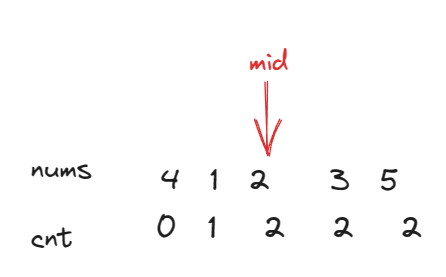②如果我们将n个数放到n+1个位置上（数的范围是1~n），这些数不重复，如果此时 cnt<=mid，则说明重复的数一定在左侧区间，因为数是在1~n这个区间选的，cnt[n]<=mid说明比n小的数不到一半（正常情况是刚好一半的），根据抽屉原理，一定是有一个比mid小的数重复了，这样才会出现cnt[n]<=mid，所以重复的数在mid的左侧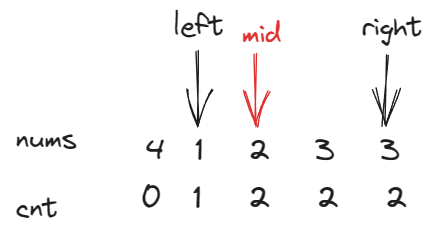③如果我们将n个数放到n+1个位置上，如果是左侧的数多了，则会导致cnt[n]>mid，此时我们可以在左侧区间寻找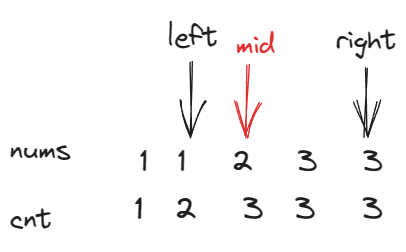温馨提示：对于所有的二分查找，边界值都是需要十分注意的，这个我在以前总结的二分查找中就已经进行了详细讲解，这里我也不在赘述了，直接给出结论，如果想要了解的，可以参考我以前写的一篇关于二分查找边界值问题的总结

1. 对于向下取整mid = (right-left)/2 + left ，如果取等 while(left<=right)，那么目标值在右right=mid-1，目标值在左left=mid+1

2. 对于向下取整mid=(right-left)/2 + left，如果不取等while(left<right)，那么目标值在右right=mid，目标值在左left=mid+1

如果取等匹配right=mid会导致死循环，如果不取等匹配right=mid-1会出现遗漏导致结果错误

/**
* @author ghp
* @title
* @description
*/
class Solution {
public int findDuplicate(int[] nums) {
int left = 1, right = nums.length - 1;
while (left < right) {
int mid = (right - left) / 2 + left;
// 计算当前小于等于mid的元素有多少个
int count = 0;
for (int i = 0; i < nums.length; i++) {
if (nums[i] <= mid){
count++;
}
}
if (count > mid){
// 比mid小的元素超过了mid个，根据抽屉原理可以知道mid左侧出现了重复元素
right = mid;
}else{
// 比mid小的元素超过了mid个，根据抽屉原理可以知道mid右侧出现了重复元素
left = mid + 1;
}
}
return left;
}
}


复杂度分析：

• 时间复杂度： O ( n l o g n ) O(nlogn)
• 空间复杂度： O ( 1 ) O(1)

其中n为数组中元素的个数

• 解法三：位运算

太强了，感兴趣的可以去看LeetCode官网，我先把前面两种解法消化吸收了

class Solution {
public int findDuplicate(int[] nums) {
int n = nums.length, ans = 0;
int bit_max = 31;
while (((n - 1) >> bit_max) == 0) {
bit_max -= 1;
}
for (int bit = 0; bit <= bit_max; ++bit) {
int x = 0, y = 0;
for (int i = 0; i < n; ++i) {
if ((nums[i] & (1 << bit)) != 0) {
x += 1;
}
if (i >= 1 && ((i & (1 << bit)) != 0)) {
y += 1;
}
}
if (x > y) {
ans |= 1 << bit;
}
}
return ans;
}
}

作者：LeetCode-Solution
链接：https://leetcode.cn/problems/find-the-duplicate-number/solution/xun-zhao-zhong-fu-shu-by-leetcode-solution/
来源：力扣（LeetCode）
著作权归作者所有。商业转载请联系作者获得授权，非商业转载请注明出处。


复杂度分析：

• 时间复杂度： O ( n l o g n ) O(nlogn)
• 空间复杂度： O ( 1 ) O(1)

其中n为数组中元素的个数

# 二叉树的序列化与反序列化

## 🔒题目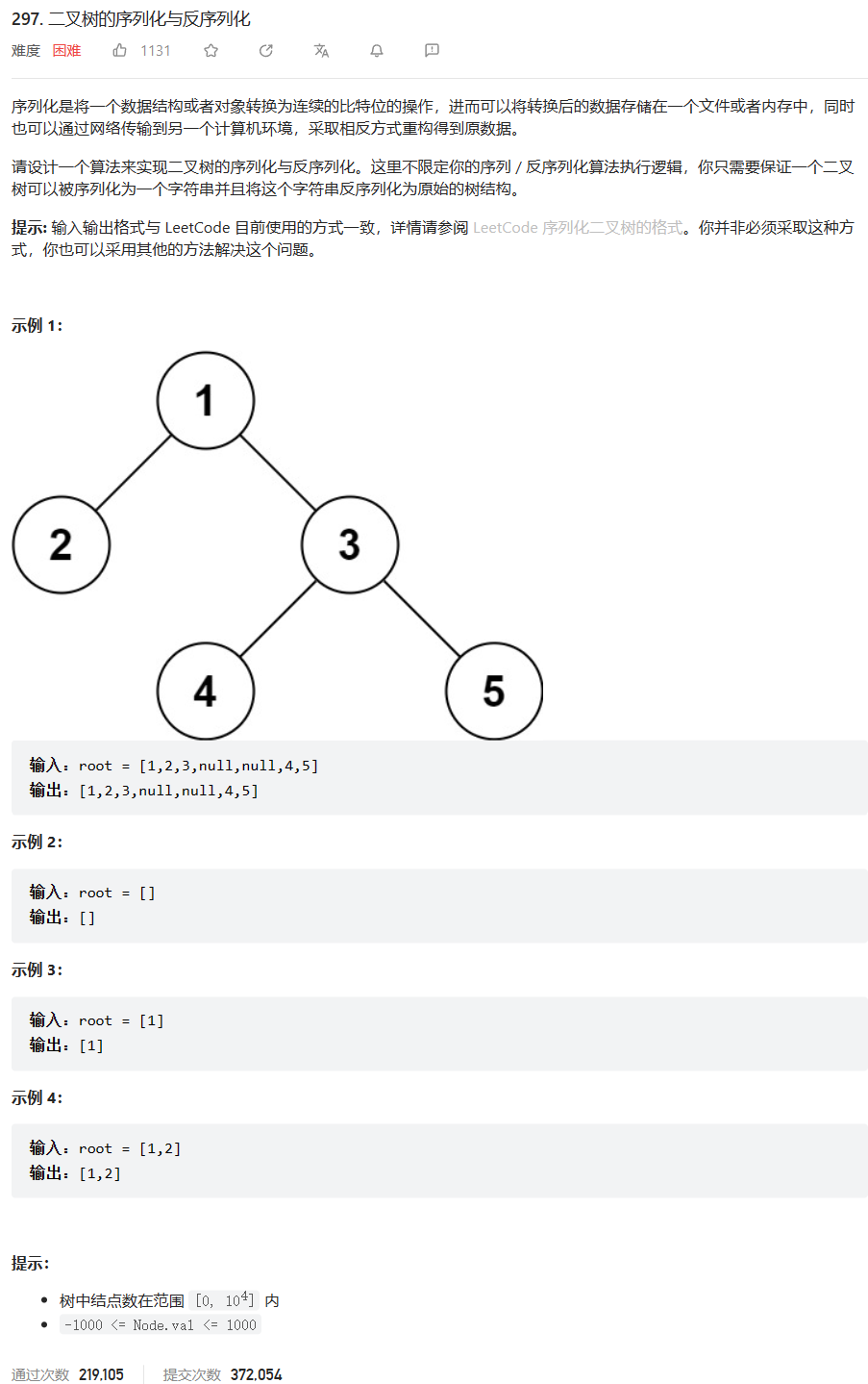## 🔑题解

• 解法一：BFS（层序遍历）

不知道为什么我第一眼看着提感觉挺简单的，直接BFS不就好了吗，结果bug频出，一眨眼一小时就过去了，经过不断的debug最终成功完成了初步代码，并最终过了😄写这题的思路也比较简答， 直接使用BFS实现层序遍历即可

如果不会层序遍历的话，可以参考这篇文章：【LeetCode热题100】打卡第29天：二叉树的层序遍历

class Codec {
public String serialize(TreeNode root) {
if (root == null) {
// 防止NPE
return null;
}
// 存储每一层的节点的值
StringBuilder ans = new StringBuilder(root.val + ",");
// BFS层序遍历所有节点，将二叉树所有节点的值转存到ans中
queue.offer(root);
while (!queue.isEmpty()) {
TreeNode pre = queue.poll();
TreeNode left = pre.left;
if (left != null) {
queue.offer(left);
}
ans.append(left == null ? "null" : left.val).append(",");
TreeNode right = pre.right;
if (right != null) {
queue.offer(right);
}
ans.append(right == null ? "null" : right.val).append(",");
}
// 删除最后一个多余的逗号
ans.deleteCharAt(ans.length() - 1);
return ans.toString();
}

public TreeNode deserialize(String data) {
if (data == null) {
// 防止NPE
return null;
}
// 将String转成List方便后续逻辑处理
String[] dataStr = data.split(",");
List<Integer> dataList = Arrays.stream(dataStr)
.map(str -> str.equals("null") ? null : Integer.valueOf(str))
.collect(Collectors.toList());
// BFS层序遍历所有节点，将层序遍历的字符串重新构建成一棵二叉树
// 将根节点加入队列中
TreeNode root = new TreeNode(dataList.get(0));
queue.offer(root);
dataList.remove(0);
while (!dataList.isEmpty()) {
TreeNode node = queue.poll();
if (dataList.get(0) != null) {
// 这里一定要判空，否则自动拆箱时会报NPE，下面那个判空也是一样的
node.left = new TreeNode(dataList.get(0));
queue.offer(node.left);
}
dataList.remove(0);
if (dataList.isEmpty()) {
// 防止NPE
break;
}
if (dataList.get(0) != null) {
node.right = new TreeNode(dataList.get(0));
queue.offer(node.right);
}
dataList.remove(0);
}
return root;
}
}


复杂度分析：

序列化

• 时间复杂度： O ( n ) O(n)
• 空间复杂度： O ( n ) O(n)

反序列化

• 时间复杂度： O ( n ) O(n)
• 空间复杂度： O ( n ) O(n)

其中 n n 为二叉树节点的个数

代码优化

对于serialize方法：

1. 每个循环只需要处理一个节点，不需要额外的变量来保存父节点

对于deserialize方法：

1. 使用整型数组代替列表，因为在循环中频繁进行插入和删除操作会导致列表的性能下降
2. 使用索引标记当前节点的位置，避免频繁调用 dataList.get() 方法
/**
* @author ghp
* @title
* @description
*/
class Codec {

public String serialize(TreeNode root) {
if (root == null) {
return null;
}
StringBuilder ans = new StringBuilder();
queue.offer(root);
while (!queue.isEmpty()) {
TreeNode node = queue.poll();
if (node != null) {
ans.append(node.val).append(",");
queue.offer(node.left);
queue.offer(node.right);
} else {
ans.append("null,");
}
}
ans.deleteCharAt(ans.length() - 1);
return ans.toString();
}

public TreeNode deserialize(String data) {
if (data == null) {
return null;
}
String[] dataStr = data.split(",");
List<Integer> dataList = Arrays.stream(dataStr)
.map(str -> str.equals("null") ? null : Integer.valueOf(str))
.collect(Collectors.toList());
TreeNode root = new TreeNode(dataList.get(0));
queue.offer(root);
int index = 1;
for (; index < dataList.size(); index += 2) {
TreeNode node = queue.poll();
if (dataList.get(index) != null) {
node.left = new TreeNode(dataList.get(index));
queue.offer(node.left);
}
if (index + 1 < dataList.size() && dataList.get(index + 1) != null) {
node.right = new TreeNode(dataList.get(index + 1));
queue.offer(node.right);
}
}
return root;
}
}

• 解法二：DFS（前序遍历）

这里主要是通过前序遍历实现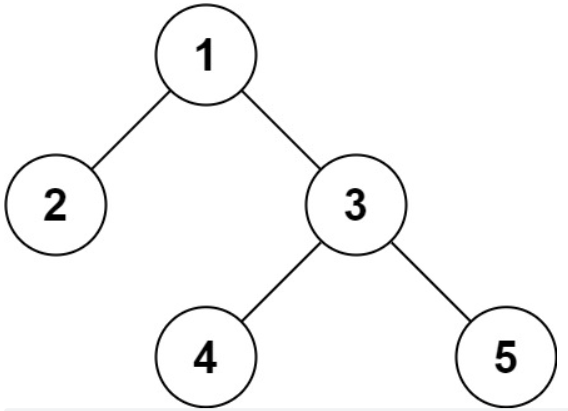1. 序列化实现比较简单，直接DFS搜索即可： [1,2,null,null,3,4,null,null,5,null,null]

2. 反序列化的时候，第一个元素为根节点，接下来都是按照前序遍历的顺序，先走左边，直到遇到 null 结束，然后换另一边

整个过程递归进行

class TreeNode {
int val;
TreeNode left;
TreeNode right;

TreeNode(int x) {
val = x;
}
}

/**
* @author ghp
* @title
* @description
*/
class Codec {

public String serialize(TreeNode root) {
StringBuilder ans = new StringBuilder();
dfs(root, ans);
ans.deleteCharAt(ans.length() - 1);
return ans.toString();
}

private void dfs(TreeNode root, StringBuilder ans) {
if (root == null) {
ans.append("null,");
return;
}
ans.append(root.val).append(",");
dfs(root.left, ans);
dfs(root.right, ans);
}

public TreeNode deserialize(String data) {
String[] dataStr = data.split(",");
// 根据前序遍历的结果构建二叉树
return buildTree(dataStr);
}

private int i = 0;
private TreeNode buildTree(String[] dataStr) {
String value = dataStr[i++];
if (value.equals("null")) {
// 防止自动拆箱导致NPE，同时也是递归结束条件
return null;
}
TreeNode node = new TreeNode(Integer.valueOf(value));
// 构建左子树
node.left = buildTree(dataStr);
// 构建右子树
node.right = buildTree(dataStr);
return node;
}
}


复杂度分析：

序列化

• 时间复杂度： O ( n ) O(n)
• 空间复杂度： O ( n ) O(n)

反序列化

• 时间复杂度： O ( n ) O(n)
• 空间复杂度： O ( n ) O(n)

其中 n n 为二叉树节点的个数

# 最长递增子序列

## 🔒题目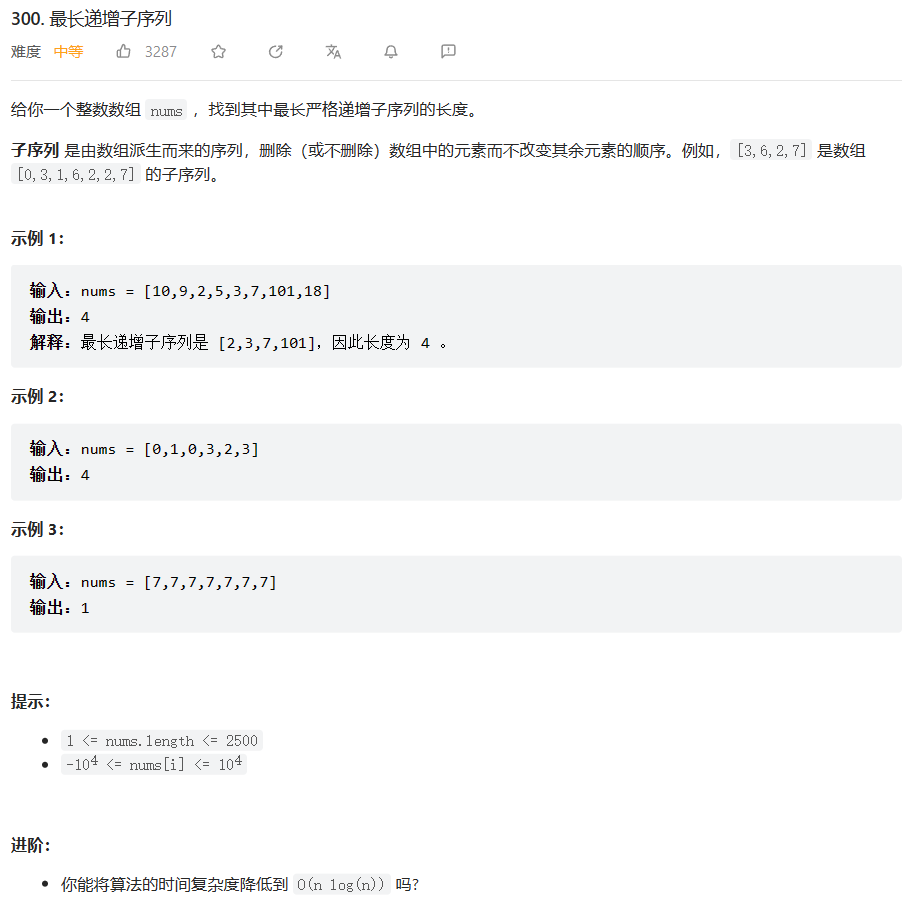## 🔑题解

• 解法一：暴力DFS（超时 22 / 54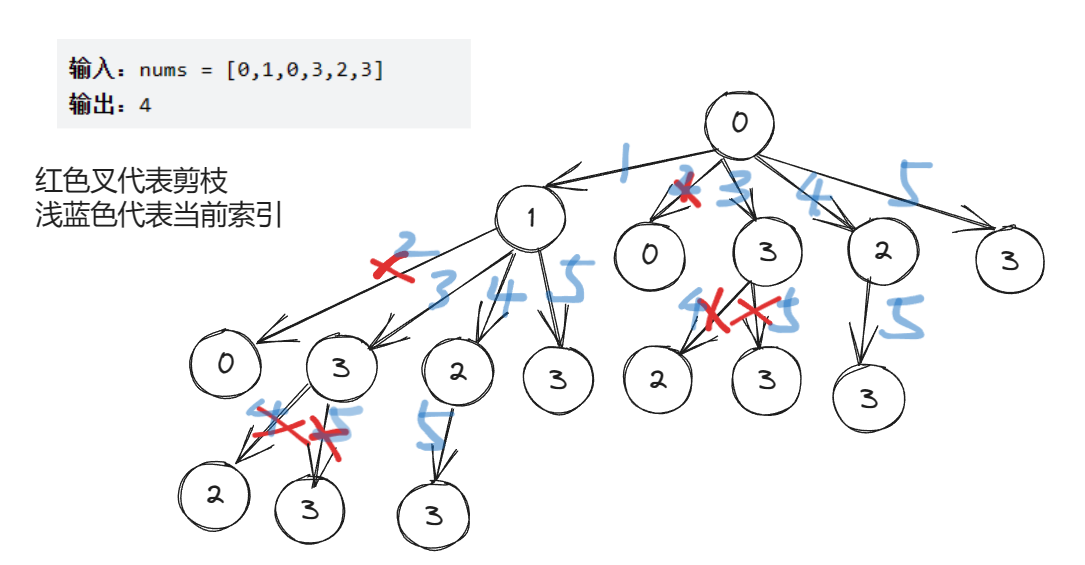PS：画的有点丑，但是能看明白就行(●ˇ∀ˇ●)

/**
* @author ghp
* @title
* @description
*/
public class Solution {
public int lengthOfLIS(int[] nums) {
// 最长递增子序列的长度
int maxLength = 0;
// DFS遍历每一个节点
for (int i = 0; i < nums.length; i++) {
int length = dfs(nums, i, Integer.MIN_VALUE);
maxLength = Math.max(maxLength, length);
}
return maxLength;
}

private int dfs(int[] nums, int index, int preLen) {
if (index == nums.length) {
// 达到数组末尾，返回长度为0
return 0;
}
int len1 = 0;
if (nums[index] > preLen) {
// 当前元素大于前一个元素，可以选择当前元素作为递增子序列的一部分
len1 = 1 + dfs(nums, index + 1, nums[index]);
}
// 不选择当前元素，继续寻找下一个递增子序列
int len2 = dfs(nums, index + 1, preLen);
// 返回选择当前元素和不选择当前元素中的较长子序列的长度
return Math.max(len1, len2);
}
}


复杂度分析：

• 时间复杂度： O ( 2 n ) O(2^n) ，每一个节点都有选和不选两种情况，所以总的来说是 2 n 2^n
• 空间复杂度： O ( l o g n ) O(logn) ，空间复杂度为递归的最大深度，最大深度是树的最大高度

其中 n n 为数组中元素的个数

代码优化：时间优化

我们可以通过记忆化搜索来大幅度提高搜索的速度，我们需要新增一个memo数组，memo[i][j]表示以第i个元素为结尾、且第j个元素为上一个结尾元素的最长递增子序列的长度。

为了新增一个记忆搜索功能，我们需要对上面代码进行一个微型改造，我们在DFS搜索时，不能像前面一样传递上一个节点的长度，而是需要传递上一个节点的索引，这样我们才能够使用memo数组对当前状态进行标记，下面的示意图是添加了记忆数组之后的搜索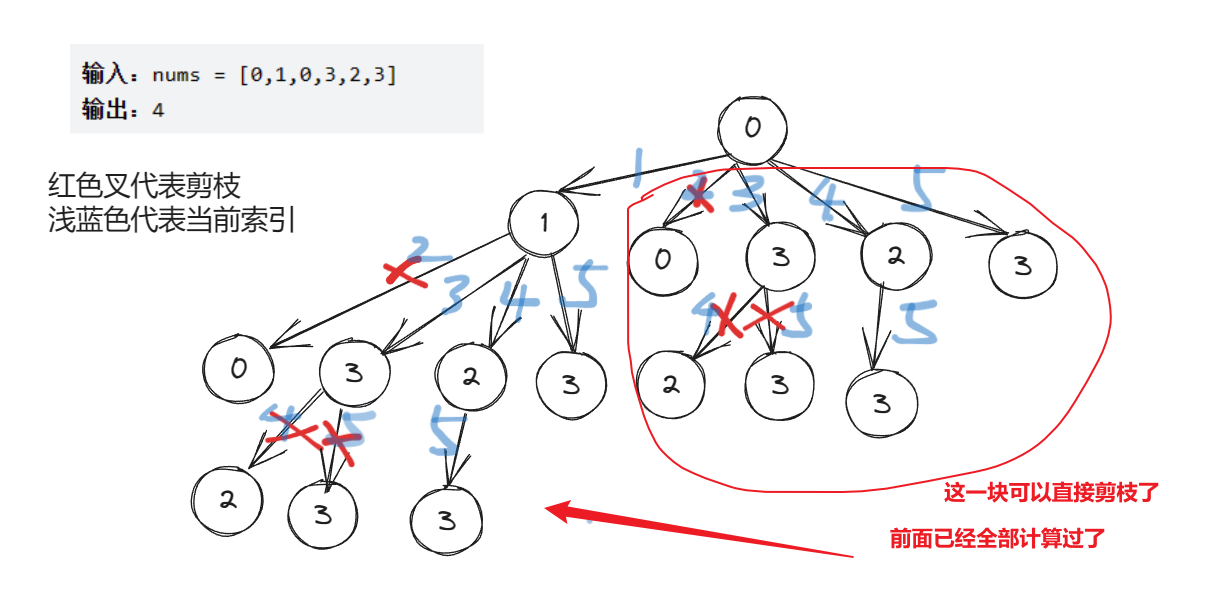通过Debug也可以看出来，每进行一次DFS，都可以直接将当前节点到其它任意节点的距离计算出来，这样就能大幅度进行剪枝了。比如上图，0到1这条路径，就可以计算出0到其它节点（1，0，3，2，3）的距离了，后面的路径0到0、0到3、0到2、0到3就不用再去重新遍历了，而是直接拿我们缓存在memo中的路径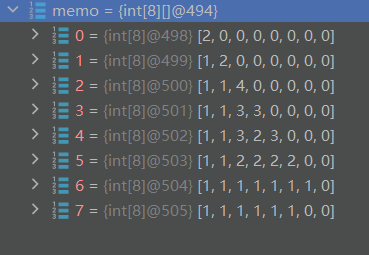public class Solution {
public int lengthOfLIS(int[] nums) {
int maxLength = 1;
// 记录节点的状态 memo[i][j]表示索引为j的节点到索引为i的节点的最长递增节点数
int[][] memo = new int[nums.length][nums.length];
// DFS搜索每一个节点
for (int i = 0; i < nums.length; i++) {
maxLength = Math.max(maxLength, dfs(nums, i, i, memo));
}
return maxLength;
}

private int dfs(int[] nums, int curIndex, int preIndex, int[][] memo) {
if (curIndex >= nums.length) {
// 后面已经没有节点了，结束搜索
return 0;
}
if (memo[curIndex][preIndex] > 0) {
// preIndex到curIndex这个状态已计算过，直接返回
return memo[curIndex][preIndex];
}
int len1 = 0;
if (preIndex == curIndex || nums[curIndex] > nums[preIndex]) {
// 当前元素大于前一个元素，可以选择当前元素作为递增子序列的一部分
len1 = 1 + dfs(nums, curIndex + 1, curIndex, memo);
}
// 不选择当前元素，继续寻找下一个递增子序列
int len2 = dfs(nums, curIndex + 1, preIndex, memo);
// 缓存preIndex到curIndex这个状态
memo[curIndex][preIndex] = Math.max(len1, len2);
// 返回选择当前元素和不选择当前元素中的较长子序列的长度
return memo[curIndex][preIndex];
}
}


记忆搜索是经典的拿时间换空间，时间复杂度虽然没有变，但是却大大缩减了搜索结果的时间，空间复杂度提高了

复杂度分析：

• 时间复杂度： O ( 2 n ) O(2^n) ，每一个节点都有选和不选两种情况，所以总的来说是 2 n 2^n
• 空间复杂度： O ( n 2 ) O(n^2) ，memo占用 n 2 n^2 的空间

其中 n n 为数组中元素的个数

备注：将 memo[curIndex][preIndex] 转换为 memo[preIndex][curIndex] 是不可行的。这是因为 preIndex 的值是固定的，是遍历时的前一个索引，而 curIndex 是在不断递增变化的。

如果我们将 memo[curIndex][preIndex] 转换为 memo[preIndex][curIndex]，则无法正确存储和查找子问题的解决方案。由于 curIndex 不断增加，我们无法准确地映射到递归调用中的子问题。

代码优化：空间优化

我们可以发现memo每进行一次DFS都只用到了一列的数据，所以我们完全可以将二维的memo压缩为一维的memo

public class Solution {
public int lengthOfLIS(int[] nums) {
int maxLength = 1;
int[] memo = new int[nums.length];
Arrays.fill(memo, 1);
for (int i = 0; i < nums.length; i++) {
maxLength = Math.max(maxLength, dfs(nums, i, memo));
}
return maxLength;
}

private int dfs(int[] nums, int curIndex, int[] memo) {
if (curIndex >= nums.length) {
return 0;
}
if (memo[curIndex] > 1) {
return memo[curIndex];
}
int maxLen = 1;
for (int i = curIndex + 1; i < nums.length; i++) {
if (nums[i] > nums[curIndex]) {
maxLen = Math.max(maxLen, 1 + dfs(nums, i, memo));
}
}
memo[curIndex] = maxLen;
return maxLen;
}
}


复杂度分析：

• 时间复杂度： O ( n 2 ) O(n^2) ，每一个节点都有选和不选两种情况，所以总的来说是 2 n 2^n
• 空间复杂度： O ( n ) O(n) ，memo占用 n n 的空间

其中 n n 为数组中元素的个数

• 解法二：动态规划

我们需要构建一个dp[i]dp[i]表示以nums[i]结尾的最长递增子序列的长度，此时我们可以知道 当前第i个节点结尾的最长递增子序列，一定是由前面的节点转移而来的，至于是前面哪一个节点，我们无法直接确定，所以此时需要遍历 前面 i+1个节点，在遍历的同时，我们不断更新当前的 dp[i]，遍历完毕，即可得到当前最大长度。

不知道为什么感觉动态规划比前面的DFS要简单多了

import java.util.Arrays;

/**
* @author ghp
* @title
* @description
*/
public class Solution {
public int lengthOfLIS(int[] nums) {
if (nums.length == 0) {
return 0;
}
int maxLength = 1;
int[] dp = new int[nums.length];
// 每一个节点自身的初始长度都是1
Arrays.fill(dp, 1);
// 遍历每一个节点
for (int i = 1; i < nums.length; i++) {
// 遍历0~i之间的节点，计算出所有以当前nums[i]结尾的最长递增子序列的长度
for (int j = 0; j < i; j++) {
if (nums[i] > nums[j]) {
dp[i] = Math.max(dp[i], dp[j] + 1);
}
}
maxLength = Math.max(maxLength, dp[i]);
}
return maxLength;
}
}


复杂度分析：

• 时间复杂度： O ( n 2 ) O(n^2)
• 空间复杂度： O ( n ) O(n)

其中 n n 为数组中元素的个数

• 解法三：动态规划+二分查找

来自：300. 最长递增子序列（动态规划 + 二分查找，清晰图解） - 最长递增子序列 - 力扣（LeetCode）

class Solution {
public int lengthOfLIS(int[] nums) {
int len = 1, n = nums.length;
if (n == 0) {
return 0;
}
int[] d = new int[n + 1];
d[len] = nums;
for (int i = 1; i < n; ++i) {
if (nums[i] > d[len]) {
d[++len] = nums[i];
} else {
int l = 1, r = len, pos = 0; // 如果找不到说明所有的数都比 nums[i] 大，此时要更新 d，所以这里将 pos 设为 0
while (l <= r) {
int mid = (l + r) >> 1;
if (d[mid] < nums[i]) {
pos = mid;
l = mid + 1;
} else {
r = mid - 1;
}
}
d[pos + 1] = nums[i];
}
}
return len;
}
}

作者：LeetCode-Solution
链接：https://leetcode.cn/problems/longest-increasing-subsequence/solution/zui-chang-shang-sheng-zi-xu-lie-by-leetcode-soluti/
来源：力扣（LeetCode）
著作权归作者所有。商业转载请联系作者获得授权，非商业转载请注明出处。


复杂度分析：

• 时间复杂度： O ( n l o g n ) O(nlogn)
• 空间复杂度： O ( n ) O(n)

其中 n n 为数组中元素的个数

# 删除无效括号

## 🔒题目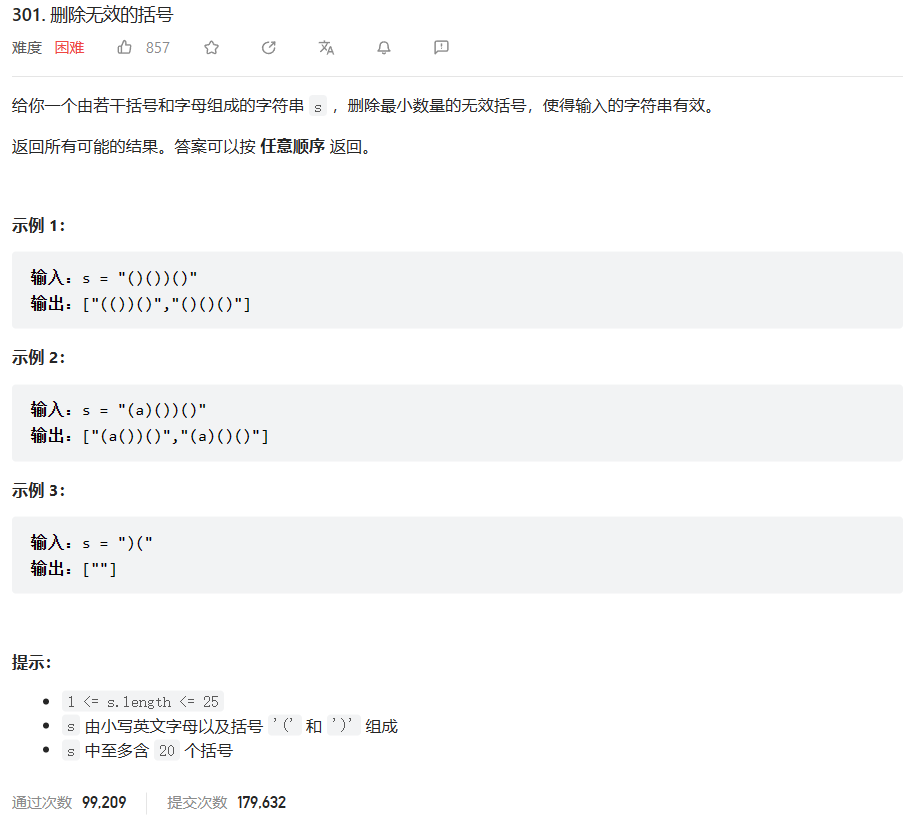## 🔑题解

• 解法一：暴力




复杂度分析：

• 时间复杂度： O ( n 2 ) O(n^2)
• 空间复杂度： O ( 1 ) O(1)

其中 n n 为数组中元素的个数

• 解法二：哈希表

这个太强了，时间复杂度直接变成 O ( n ) O(n) ，它是利用Map的Key不能重复的特性，来判断元素是否符合要求。




复杂度分析：

• 时间复杂度： O ( n ) O(n)
• 空间复杂度： O ( n ) O(n)

其中 n n 为数组中元素的个数

• 9种方法（可能是目前最全的），拓展大家思路 - 寻找重复数 - 力扣（LeetCode）
• 使用「二分查找」搜索一个有范围的整数（结合「抽屉原理」） - 寻找重复数 - 力扣（LeetCode）
• 【图解】dfs + bfs + 后序遍历 + 其他思路 - 二叉树的序列化与反序列化 - 力扣（LeetCode）# 【LeetCode热题100】打卡第44天：倒数第30~25题

### leetcode 491. 递增子序列

2023.7.23 本题本质上也是要选取递归树中的满足条件的所有节点&#xff0c;而不是选取叶子节点。 故在将符合条件的path数组放入ans数组后&#xff0c;不要执行return。 还一点就是这个数组不是有序的&#xff0c;并且也不能将它有序化&#xff0c;所以这里的去重操作不能和之前…

### 2023-07-27：最长可整合子数组的长度， 数组中的数字排序之后，相邻两数的差值是1， 这种数组就叫可整合数组。 给定一个数组，求最长可整合子数组的长度。

2023-07-27&#xff1a;最长可整合子数组的长度&#xff0c; 数组中的数字排序之后&#xff0c;相邻两数的差值是1&#xff0c; 这种数组就叫可整合数组。 给定一个数组&#xff0c;求最长可整合子数组的长度。 答案2023-07-27&#xff1a; 算法maxLen的过程如下&#xff…

### 【体系认证】ISO27701 隐私信息管理体系

1 认证定义 ISO/IEC 27701 隐私信息管理体系是ISO国际标准化组织和IEC国际电工委员会联合发布的隐私信息管理体系国际标准&#xff0c;它是对SO27001信息安全管理体系的扩展&#xff0c;在全球普遍受到认可&#xff0c;且具国际权威性。 ISO/IEC27701通过对隐私保护的控制对…

### 安全杂记 - js中的this关键字

javascript里什么是this this是js中的一个关键字&#xff0c;它是函数在运行时生成的一个内部对象&#xff0c;是属性和方法。 this就是属性或方法“当前”所在的对象&#xff0c;也就是调用函数的那个对象 this的使用场合 1.函数调用 <script>var a100;function test…

### 机器学习深度学习——感知机

&#x1f468;‍&#x1f393;作者简介&#xff1a;一位即将上大四&#xff0c;正专攻机器学习的保研er &#x1f30c;上期文章&#xff1a;机器学习&&深度学习——softmax回归的简洁实现 &#x1f4da;订阅专栏&#xff1a;机器学习&&深度学习 希望文章对你们…

### OpenAI宣布安卓版ChatGPT正式上线；一站式 LLM底层技术原理入门指南

&#x1f989; AI新闻 &#x1f680; OpenAI宣布安卓版ChatGPT正式上线 摘要&#xff1a;OpenAI今日宣布&#xff0c;安卓版ChatGPT已正式上线&#xff0c;目前美国、印度、孟加拉国和巴西四国的安卓用户已可在谷歌Play商店下载&#xff0c;并计划在下周拓展到更多地区。Chat…